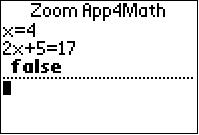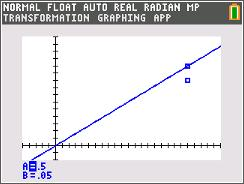### Boats in Motion

Students explore the motion of a boat going up and down the river. They will be instructed to solve the resulting system of equations algebraically and graphically.
•TI-84 Plus CE
•TI-84 Plus C Silver Edition
• TI-84 Plus Silver Edition
• TI-84 Plus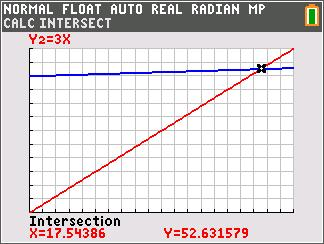Students will work through a scenario of a business venture involving washing dogs.
•TI-84 Plus CE
•TI-84 Plus C Silver Edition
• TI-84 Plus Silver Edition
• TI-84 Plus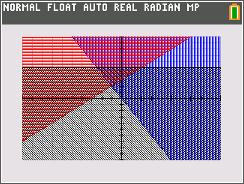### Tri This!

Students investigate linear equations that form a triangle and determine which vertex is a solution to a system of equations.
•TI-84 Plus CE
•TI-84 Plus C Silver Edition
• TI-84 Plus Silver Edition
• TI-84 Plus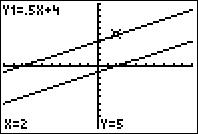### All On The Line

Students enter points into a spreadsheet and graph equations to help solve linear systems.
•TI-84 Plus CE
•TI-84 Plus C Silver Edition
• TI-84 Plus Silver Edition
• TI-84 Plus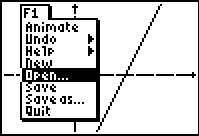### How Many Solutions?

In this activity, students graph systems of linear functions to determine the number of solutions. In the investigation, students are given one line and challenged to draw a second line that creates a system with a particular number of solutions.
•TI-84 Plus CE
•TI-84 Plus C Silver Edition
• TI-84 Plus Silver Edition
• TI-84 Plus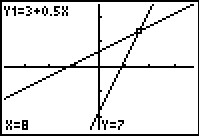### Understanding Solutions of Systems Using Tables and Graphs

Students represent and analyze mathematical situations and structures using algebraic symbols.
•TI-84 Plus CE
•TI-84 Plus C Silver Edition
• TI-84 Plus Silver Edition
• TI-84 Plus